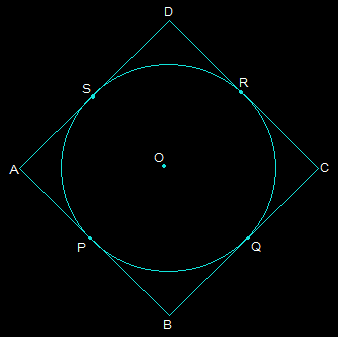Guru

# Prove that the parallelogram circumscribing a circle is a rhombus. Q.11

• 1

How i prove the parallelogram of question no.11 of ncert class 10th book of exercise 10.2, please give me the simple way for to prove the parallelogram  Prove that the parallelogram circumscribing a circle is a rhombus.

Share

1. Consider a parallelogram ABCD which is circumscribing a circle with a center O. Now, since ABCD is a parallelogram, AB = CD and BC = AD.From the above figure, it is seen that,

(i) DR = DS

(ii) BP = BQ

(iii) CR = CQ

(iv) AP = AS

These are the tangents to the circle at D, B, C, and A respectively.

DR+BP+CR+AP = DS+BQ+CQ+AS

By rearranging them we get,

(BP+AP)+(DR+CR) = (CQ+BQ)+(DS+AS)

Again by rearranging them we get,